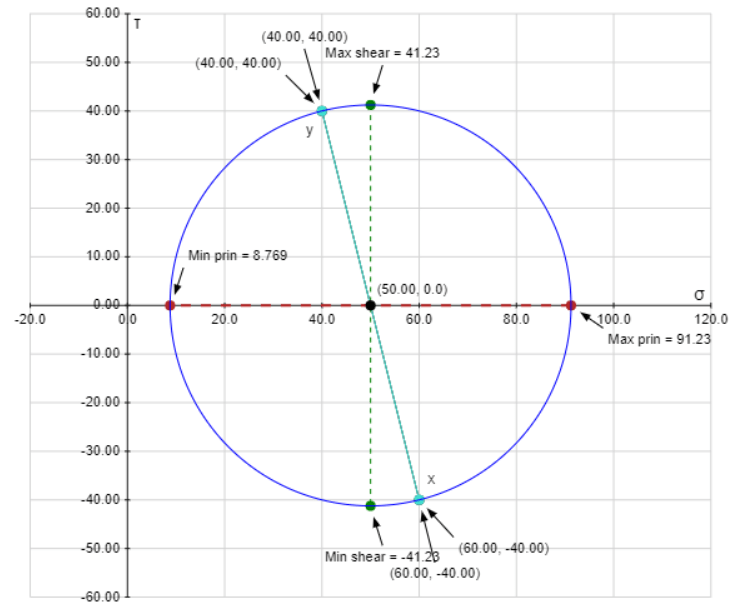# At a point in an elastic material under strain, the stresses on the three mutually perpendicular planes are as follows: A normal tensile stress of 50 N/mm2 and shear stress of 50 N/mm2 on one plane and a normal tensile force of 40 N/mm2 and a complimentary shear stress of 50 N/mm2 on another plane. Find the following using Mohr circle only (take 5 N/mm2 = 2 cm) a. The principal stresses and principal planes. b. The maximum shear stress and its plane. c. The normal and shear stress on a plane inclined at an angle of 30° to major principal plane

Question-AnswerCategory: Strength of MaterialsAt a point in an elastic material under strain, the stresses on the three mutually perpendicular planes are as follows: A normal tensile stress of 50 N/mm2 and shear stress of 50 N/mm2 on one plane and a normal tensile force of 40 N/mm2 and a complimentary shear stress of 50 N/mm2 on another plane. Find the following using Mohr circle only (take 5 N/mm2 = 2 cm) a. The principal stresses and principal planes. b. The maximum shear stress and its plane. c. The normal and shear stress on a plane inclined at an angle of 30° to major principal plane

At a point in an elastic material under strain, the stresses on the three mutually perpendicular
planes are as follows:
A normal tensile stress of 60 N/mm2
and shear stress of 50 N/mm2 on one plane and a normal tensile force of 40 N/mm2 and a complimentary shear stress of 50 N/mm2 on another plane. Find the following using Mohr circle only (take 5 N/mm2 = 2 cm)
a. The principal stresses and principal planes.
b. The maximum shear stress and its plane.
c. The normal and shear stress on a plane inclined at an angle of 30°
to major principal plane

Step 1
Given:
Normal tensile and shear stress on one plane are 60 N/mm2 and 40 N/mm2.
Normal tensile and shear stress on another plane are 40 N/mm2 and 40 N/mm2.
Step 2
Calculation:
The plane stress problem is shown below:Take 5 N/mm2 = 1 cm and draw the Mohr’s circle as shown below:
Step 3(a)
From the above Mohr’s circle, the maximum and minimum principal stress with angles are:
F1=91.23 Mpa at angle θ =37.98°
F2=8.76 Mpa at angle θ = 128.0°
(b)
From the above Mohr’s circle, the maximum shear stress with angle is:
τmax = 91.2 Mpa at angle θ =82.98°
(c)
The normal and shear stress at 30 deg from the maximum principal stress is calculated as follows:In triangle AOB,
sin30=AB/OA
sin30=AB/R
sin30=AB/41.231
AB==20.6155 Mpa
And,
cos30=OB/OA
cos30°=OB/R
cos30°=OB/41.231
OB=σn=35.707 Mpa

Thus, the normal and shear stresses are 35.707 Mpa and 20.6155 Mpa.